NCERT Solutions: Basic Geometrical Ideas- 1

# NCERT Solutions: Basic Geometrical Ideas- 1 Notes | Study Mathematics (Maths) Class 6 - Class 6

## Document Description: NCERT Solutions: Basic Geometrical Ideas- 1 for Class 6 2022 is part of Mathematics (Maths) Class 6 preparation. The notes and questions for NCERT Solutions: Basic Geometrical Ideas- 1 have been prepared according to the Class 6 exam syllabus. Information about NCERT Solutions: Basic Geometrical Ideas- 1 covers topics like Exercise 4.1, Exercise 4.2, Exercise 4.3 and NCERT Solutions: Basic Geometrical Ideas- 1 Example, for Class 6 2022 Exam. Find important definitions, questions, notes, meanings, examples, exercises and tests below for NCERT Solutions: Basic Geometrical Ideas- 1.

Introduction of NCERT Solutions: Basic Geometrical Ideas- 1 in English is available as part of our Mathematics (Maths) Class 6 for Class 6 & NCERT Solutions: Basic Geometrical Ideas- 1 in Hindi for Mathematics (Maths) Class 6 course. Download more important topics related with notes, lectures and mock test series for Class 6 Exam by signing up for free. Class 6: NCERT Solutions: Basic Geometrical Ideas- 1 Notes | Study Mathematics (Maths) Class 6 - Class 6
 1 Crore+ students have signed up on EduRev. Have you?

Exercise 4.1

Q1. Use the figure to name: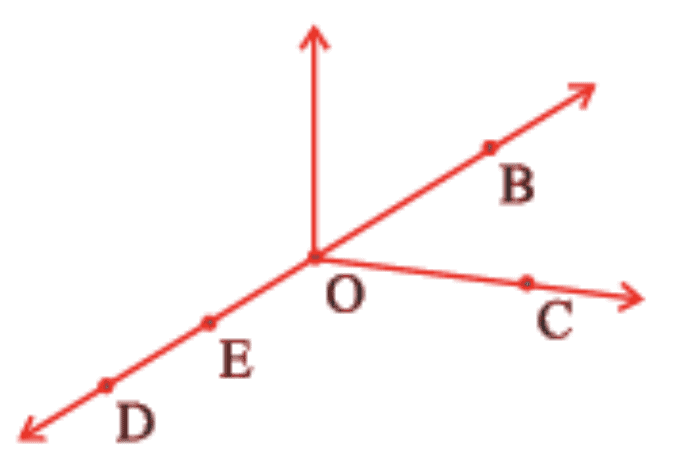(a) Five points
(b) A line
(c) Four rays
(d) Five line segments
Ans:

 (a) Five points are: O, B, C, D, E (b) A line: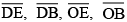(c) Four rays: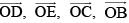(d) Four line segments: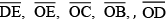Q2. Name the line given in all possible (twelve) ways, choosing only two letters at a time from the four given.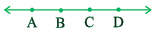Ans: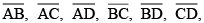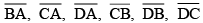Q3. Use the figure to name: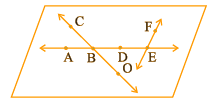(a) Line containing point E.
Ans: A line containing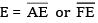(b) Line passing through A.
Ans: A line passing through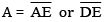(c) Line on which O lies.
Ans: A line on which O lies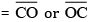(d) Two pairs of intersecting lines.
Ans: Two pairs of intersecting lines are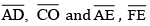Q4. How many lines can pass through

(a) one given point?
Ans: Infinite number of lines can pass through one given point.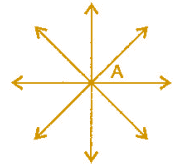(b) Two given points?
Ans: Only one line can pass through two given points.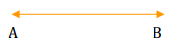Q5. Draw a rough figure and label suitably in each of the following cases:
(a) Point P lies on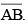.
(b)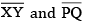intersect at M.
(c) Line contains E and F but not D.
(d)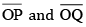meet at O.
Ans: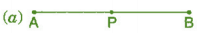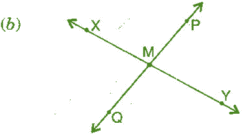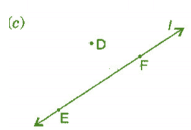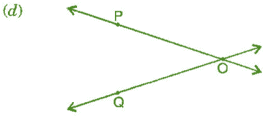Q6. Consider the following figure of line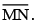Say whether following statements are true or false in context of the given figure.

(a) Q, M, O, N, P are points on the line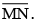Ans: True

(b) M, O, N are points on a line segmentAns: True

(c) M and N are end points of line segmentAns: True

(d) O and N are end points of line segment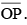Ans: False

(e) M is one of the end points of line segment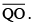Ans: False

(f) M is point on rayAns: False

(g) Ray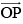is different from rayAns: True

(h) Rayis same as ray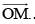Ans: False

(i) Rayis not opposite to rayAns: False

(j) O is not an initial point of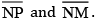Ans: False

Exercise 4.2

Q1. Classify the following curves as (i) Open or (ii) Closed.

(a)Ans: Open curve

(b)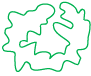Ans: Closed curve

(c)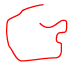Ans: Open curve

(d)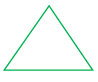Ans: Closed curve

(e)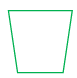Ans: Closed curve

Q2. Draw rough diagrams to illustrate the following:

(a) Open curve
Ans: Open curves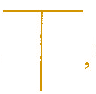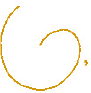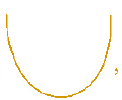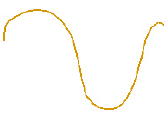(b) Closed curve
Ans: Closed curves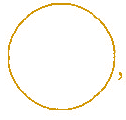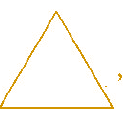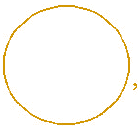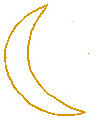Q3. Draw any polygon and shade its interior.
Ans: Polygon ABCDE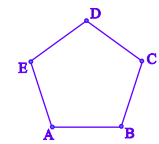Q4. Consider the given figure and answer the questions:
(a) Is it a curve?
(b) Is it closed?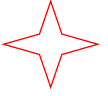Ans: (a) Yes, it is a curve.
(b) Yes, it is closed.

Q5. Illustrate, if possible, each one of the following with a rough diagram:

(a) A closed curve that is not a polygon.
Ans: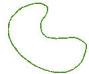(b) An open curve made up entirely of line segments.
Ans: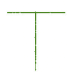(c) A polygon with two sides.
Ans: Polygon with two sides cannot be draw.

Exercise 4.3

Q1. Name the angles in the given figure.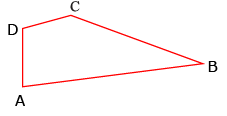Ans: There are four angles in given figure:
∠ ABC, ∠ CDA, ∠ DAB, ∠ DCB

Q2. In the given diagram, name the point (s)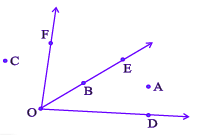(a) In the interior of ∠DOE
Ans: Point interior of ∠DOE : A

(b) In the exterior of ∠EOF
Ans: Points exterior of ∠EOF: C, A, D

(c) On ∠EOF
Ans: Points on ∠EOF: E, O, B, F

Q3. Draw rough diagrams of two angles such that they have

(a) One point in common.
Ans: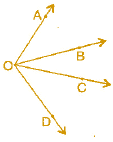(b) Two points in common.
Ans: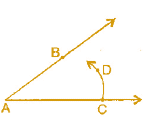(c) Three points in common.
Ans: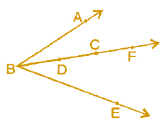(d) Four points in common.
Ans: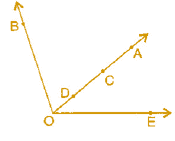(e) One ray in common.
Ans: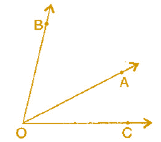The document NCERT Solutions: Basic Geometrical Ideas- 1 Notes | Study Mathematics (Maths) Class 6 - Class 6 is a part of the Class 6 Course Mathematics (Maths) Class 6.
All you need of Class 6 at this link: Class 6

## Mathematics (Maths) Class 6

167 videos|275 docs|43 tests
 Use Code STAYHOME200 and get INR 200 additional OFF

## Mathematics (Maths) Class 6

167 videos|275 docs|43 tests

Track your progress, build streaks, highlight & save important lessons and more!

,

,

,

,

,

,

,

,

,

,

,

,

,

,

,

,

,

,

,

,

,

;